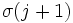Transpositions generate the finitary symmetric group

This article states and proves a fact about a particular group, or kind of group, (i.e., finitary symmetric group) having a particular generating set (or kind of generating set), i.e., where the generators are elements of the type/form: transposition
View other facts about generating sets for particular groups

Statement

For a finite set

The Symmetric group (?) on a finite set is generated by the Transposition (?)s in it. A transposition is a permutation that interchanges two elements and leaves all the others fixed; in other words, it is a cycle of length two.

For an infinite set

The Finitary symmetric group (?) on any set is generated by the transpositions in it. A transposition is a permutation that interchanges two elements and leaves all the others fixed; in other words, it is a cycle of length two.

Applications

This result proves, for instance, that the symmetric group on a finite set, and more generally, any finitary symmetric group, is a group generated by involutions -- it is generated by elements of order two.

Related facts

Related facts about generating sets for permutation groups

For the symmetric group on a set of size$n$, the transpositions form a generating set of size$\binom{n}{2}$, the transpositions of adjacent elements form a generating set of size$n - 1$, and the transpositions involving one particular element also form a generating set of size$n - 1$. Similar results hold for arbitrary subgroups of the symmetric group:

• Sims filter gives a procedure for starting with any generating set of a subgroup of the symmetric group on$n$ elements, and producing a generating set of size at most$\binom{n}{2}$. A generating set obtained by this filter has some special properties, and such a generating set is termed a Sims-reduced generating set.
• Jerrum's filter gives a procedure for starting with any generating set of a subgroup of the symmetric group on$n$ elements, and producing a generating set of size at most$n - 1$. A generating set obtained by this filter has some special properties, and such a generating set is termed a Jerrum-reduced generating set.

In terms of IAPS theory

In terms of IAPS theory, this translates to saying that the transposition on a two-element set forms a one-element permutatively generating set for the permutation IAPS.

Proof for a finite set

Some of the proofs given here show some of the additional facts mentioned in the Related facts section: that a permutation that moves$n$ elements can be expressed as a product of at most$n-1$ transpositions. Other proofs show that, in fact, we don't even need all the transpositions: we only need all the transpositions involving one specific element. Other proofs show that if we order the elements of a finite set, we do not need all the transpositions: we only need transpositions between adjacent elements in the ordering.

Proof using the cycle decomposition

This proof uses the cycle decomposition theorem for permutations. This theorem states that every finitary permutation of a group can be expressed as a product of disjoint cycles. Thus, it suffices to show that every cycle can be expressed as a product of transpositions.

Consider a cycle:$(a_1,a_2,\dots,a_r)$.

We claim that (using the left action convention) this cycle is a product of transpositions:$(a_1,a_r)(a_1,a_{r-1})\dots (a_1,a_2)$.

Let's prove this. For$j \ne 1,r$,$a_j$ first occurs in the transposition$(a_1,a_j)$, where it gets sent to$a_1$. Next is the transposition$(a_1,a_{j+1})$, sending it to$a_{j+1}$.$a_{j+1}$ does not get moved any further. Thus,$a_j$ gets sent to$a_{j+1}$.

For$j = 1$, the first transposition sends it to$a_2$, and$a_2$ does not get moved any further.

For$j = r$, the last transposition sends it to$a_1$.

Thus, the product of these transpositions has the same effect on every element as the prescribed$r$-cycle.

Note that since we need$r-1$ transpositions for a$r$-cycle, it is clear that for a permutation that moves$n$ elements, we need at most$n-1$ transpositions.

Proof using sorting algorithms

This proof works for the symmetric group on finite sets. The result for the finitary symmetric group on an infinite set follows from the fact that any finitary permutation is contained in the symmetric group on a finite subset.

The idea behind these proofs is to show that given any permutation, we can perform multiply it by transpositions, one after the other, to arrive at the identity permutations. Doing the inverses of these operations would thus express the given permutation as the product of transpositions.

These proofs simplify further using induction. Here, we assume that every permutation on a set of size$n-1$ is a product of transpositions, and we show that a permutation on a set of size$n$ can be expressed as a product of transposition, times a permutation on a set of size$n-1$.

• The selection sort proof: Here, given a permutation$\sigma$ on a set$\{ a_1, a_2, \dots, a_n \}$, we consider a transposition$\tau = (a_n, \sigma(a_n))$. The product permutation$\tau\sigma$ sends$a_n$ to itself, and is thus a permutation on the subset$\{ a_1, a_2, \dots, a_{n-1} \}$. Induction now applies. Note that this algorithm shows that every permutation moving$n$ elements can be expressed as a product of at most$n-1$ transpositions.
• The bubble sort proof: Here, given a permutation$\sigma$ on a set$\{ 1,2, \dots, n\}$, we do the following: traversing the list, right-multiply by the transposition$(j,j+1)$ if the index of$\sigma(j)$ is greater than$\sigma(j+1)$. This is effectively the same as left-multiplying by$(\sigma(j),\sigma(j+1))$, or equivalently, it is the same as swapping the values in positions$j$ and$j + 1$. At the end of one phase of this,$\sigma(n) = n$ (the largest entry bubbles to the bottom) so$\sigma$ is a permutation on$n - 1$ elements. Note that this proof uses a smaller generating set (the transpositions of adjacent elements only) but may require longer products.